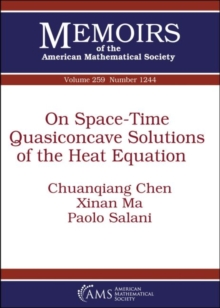On Space-Time Quasiconcave Solutions of the Heat Equation Paperback / softback

Part of the Memoirs of the American Mathematical Society series

Description

In this paper the authors first obtain a constant rank theorem for the second fundamental form of the space-time level sets of a space-time quasiconcave solution of the heat equation.

Utilizing this constant rank theorem, they obtain some strictly convexity results of the spatial and space-time level sets of the space-time quasiconcave solution of the heat equation in a convex ring.

To explain their ideas and for completeness, the authors also review the constant rank theorem technique for the space-time Hessian of space-time convex solution of heat equation and for the second fundamental form of the convex level sets for harmonic function.

Information

• Format: Paperback / softback
• Pages: 83 pages
• Publisher: American Mathematical Society
• Publication Date:
• Category: Differential calculus & equations
• ISBN: 9781470435240

£79.95

£79.25

on all orders

Pick up orders

from local bookshops

£79.95

£79.25

£79.95

£79.25

£79.95

£79.25

£79.95

£79.25